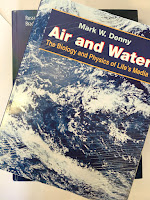## Friday, June 24, 2022

### Specific Heat Capacity

One of the most important parameters in thermodynamics is the specific heat capacity. In Chapter 3 of Intermediate Physics for Medicine and Biology, Russ Hobbie and I define the specific heat capacity in this way:
Consider a system into which a small amount of heat Q flows. In many cases the temperature of the system rises [by an amount ΔT]… The heat capacity C of the system is defined as

C = Q/ΔT .                 (3.39)

Heat capacity has units of J K-1. It depends on the size of the object and the substance it is made of. The specific heat capacity, c, is the heat capacity per unit mass (J K−1 kg−1).Air and Water,by Mark Denny.
In Air and Water: The Biology and Physics of Life's Media, Mark Denny compares the specific heat capacity of life’s two most important substances.
The specific heat of air is 1006 J kg−1 K−1, a typical value for a gas… Water, however, … has a very high specific heat for a liquid… about 4200 J kg−1 K−1… It thus takes about four times as much heat to raise the temperature of a kilogram of water one degree as it does to raise the temperature of an equal mass of air.
A factor of four is significant, but frankly I would have expected an even bigger difference. The reason for the somewhat similar values of the specific heat capacity for air and water is that we are comparing heat capacity per unit mass. It may be more intuitive to compare heat capacity per unit volume. To convert from per kilogram to per cubic meter you must multiply the specific heat capacity per unit mass by the mass per unit volume, which is just the density, ρ. The density of air and water are very different. Air has a density of about 1.2 kg m−3, whereas water has a density of 1000 kg m−3.

We can express the specific heat capacity per unit volume as the product c times ρ. For air cρ is 1207 J K−1 m−3 but for water cρ is 4,200,000 J K−1 m−3. So water has a vastly higher specific heat capacity compared to air when expressed as per unit volume.

Denny says the same thing this way:
The relative similarity of specific heat between air and water can be misleading, however, because specific heat is measured on per-mass basis. One cubic meter of air weighs only about 1.2 kg, while a cubic meter of water weighs 1000 kg. It thus takes about 3500 times as much heat to raise the temperature of a given volume of water one degree as it does to raise the temperature of the same volume of air.
For similar volumes of air and water in thermal equilibrium, the heat stored in the air is negligible compared to that stored in the water. Biological tissue is mostly water, so that means air holds a lot less heat than tissue, per unit volume. This has implications for biological processes, such as heat exchange between air and tissue in the lungs.